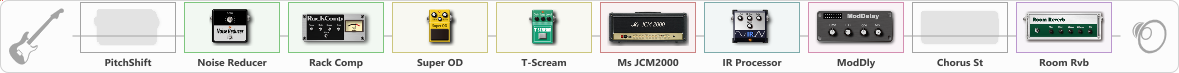# JCM2000

Discussion in 'ToneLib-GFX presets' started by Summers, May 10, 2023.

1. JCM2000

Preset name: jcm2000

Hope you enjoy

Effects chain:Effect: "PitchShift" (Modulation / Sfx), active - "no"
{
"Pitch" = -1
"Fine" = 0
"Tracking" = 34
"Direct" = 0
"Effect" = 100
}

Effect: "Noise Reducer" (Dynamics / Filter), active - "yes"
{
"Sens" = 100
"Mode" = Soft
}

Effect: "Rack Comp" (Dynamics / Filter), active - "yes"
{
"Threshold (dB)" = -20
"Ratio" = 6
"Attack" = Fast
"Release (ms)" = 42
"Knee" = 49
"Level (dB)" = 1
}

Effect: "Super OD" (Overdrive / Distortion), active - "yes"
{
"Drive" = 41
"Tone" = 75
"Level" = 22
}

Effect: "T-Scream" (Overdrive / Distortion), active - "yes"
{
"Drive" = 59
"Tone" = 65
"Level" = 100
}

Effect: "Ms JCM2000" (Amp simulators), active - "yes"
{
"Gain" = 73
"Bass" = 44
"Middle" = 50
"Treble" = 71
"Presence" = 46
"Master" = 66
"Level (dB)" = 4
}

Effect: "IR Processor" (Cabinets), active - "yes"
{
"IR" = Mesa 4x12 SPKR 1 position 2
"Low Cut (Hz)" = 100
"Hi Cut (kHz)" = 20.0
"Mix" = 100
"Level (dB)" = 0
}

Effect: "ModDly" (Delay), active - "yes"
{
"Time" = 457
"Feedback" = 45
"Tone" = 70
"Speed" = 1.6
"Mix" = 35
}

Effect: "Chorus St" (Modulation / Sfx), active - "no"
{
"Speed" = 1.9
"Depth" = 31
"Center" = 4.4
"Mix" = 40
}

Effect: "Room Rvb" (Reverberation), active - "yes"
{
"Time" = 4.5
"PreDelay" = 0
"LoDamp" = 46
"HiDamp" = 25
"Mix" = 31
}

Note: You will need to download and install the ToneLib-GFX software to use the preset.

#### Attached Files:

• ###### JCM2000.tlgfx
File size:
29.2 KB
Views:
545
AtlanticKingfish likes this.